# HUB75 LED Display Driver

This is custom 5x5cm Arduino UNO board that allows simple connection to HUB75 interface LED displays.

BeginnerFull instructions provided2 hours16,186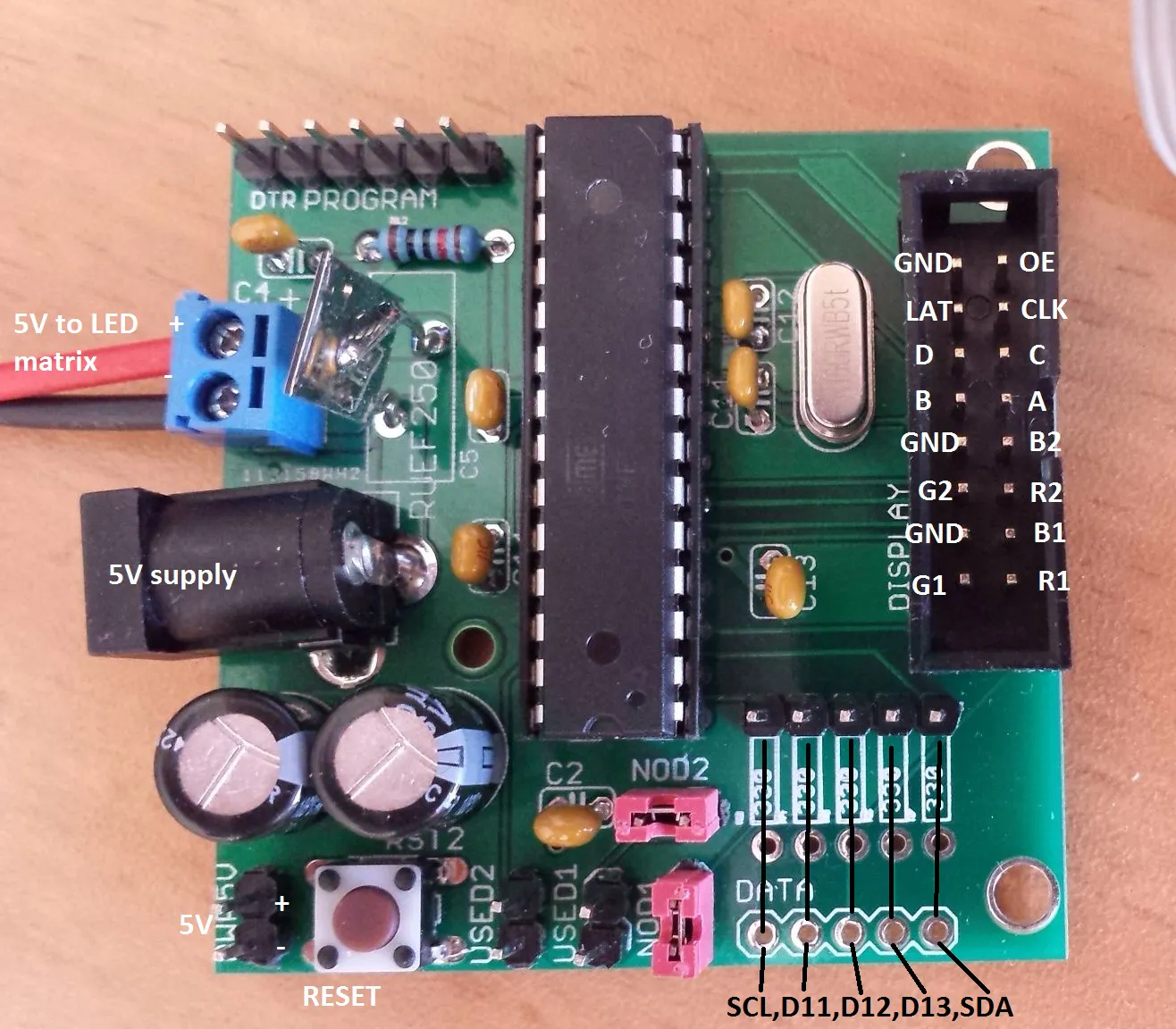## Things used in this project

### Hardware components

 ATmega328 with Arduino Optiboot (Uno)
×1Resistor 10k ohm
×1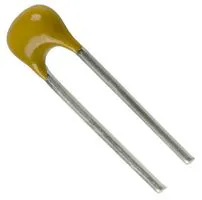Capacitor 22 pF
×2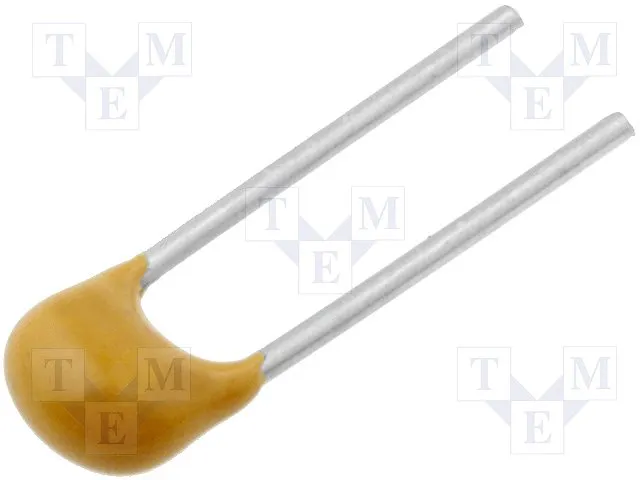Capacitor 100 nF
×5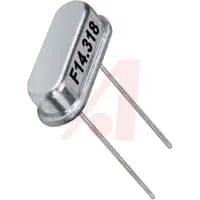16 MHz Crystal
×1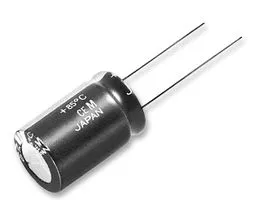Capacitor 470 µF
×2
 DC Barrel Power Jack/Connector
×1
 Two pins screw terminal block 3.5mm pitch
×1SparkFun Pushbutton switch 12mm
×1
 DIP Socket, 28 Contacts
×1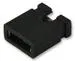Generic Jumper (0.1")
×2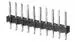Male Header 40 Position 1 Row (0.1")
×1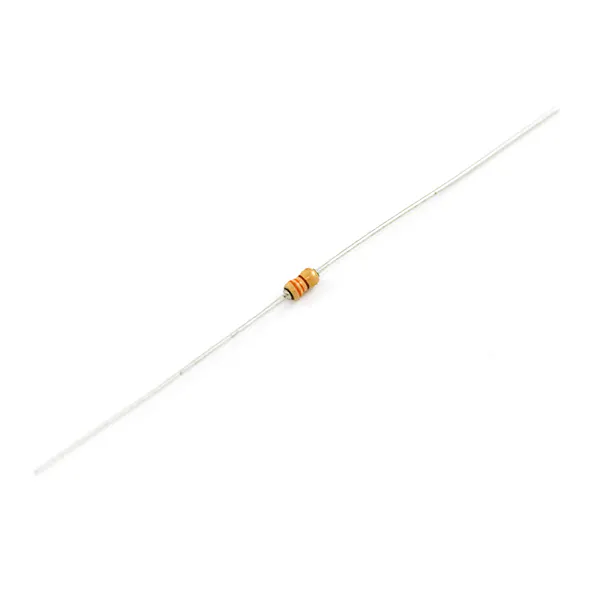Resistor 330 ohm
×5
 RUEF250U FUSE
×1
 Ribbon Cable Connector 2x8
×1

### Hand tools and fabrication machinesSoldering iron (generic)

## Code

### My library to drive 16x32 led matrix zip

C/C++
`No preview (download only).`

### Clock example

C/C++
`No preview (download only).`

### HUB75driver class

C/C++
This class allows to drive 16x32 matrix
```// Library to drive HUB75 interface 32x16 LED matrix using Arduino Uno
// RGBmatrixPanel Arduino library for Adafruit
// Adafruit Industries used as example, and couple of lines of code taken from it
// Pins usaga compatible with Adafruit RGBmatrixPanel Arduino library
// Pins mapping for HUB75 interface A A0,B A1,C A2,R1 2,R2 5,B1 4,B2 7,G1 3,G2 6,LAT A3,CLK 8,OE 9

#include "HUB75driver.h"

static HUB75driver *activePanel = NULL;

HUB75driver::HUB75driver()
{

}

uint8_t HUB75driver::init(boolean dbuf,boolean extra_dim)
{
/*Initialisation of matrix
if dbuf=true double buffering used
extra_dim alows additional dimming. brightness will reduce by half
in this case swapBuffers() function need to be called to update picture
Pin mapping:
A A0,B A1,C A2,R1 2,R2 5,B1 4,B2 7,G1 3,G2 6,LAT A3,CLK 8,OE 9
*/

use_dbuf = dbuf;
swap_needed = 0;
cp_back_to_front = 0;
half_brightness = extra_dim;
activePanel = this; // For interrupt hander

DDRD = DDRD | B11111100;//sets pins 2 to 7 as outputs without changing the value of pins 0 & 1, which are RX & TX
DDRC = DDRC | B00001111;//Set pins A0,A1,A2,A3 as output
DDRB = DDRB | B00000011;//Set pins 8,9 as output
PORTD = PORTD & B00000011;//Zero to outputs 2 to 7
PORTC = PORTC & B11111000;//Zero to A0,A1,A2
PORTB = (PORTB & B11111110) | B00000010;//* - CLK low,9 OE high

// Allocate and initialize matrix buffer:
buffsize = 32 * 8 * 3; // x3 = 3 bytes holds 4 planes "packed"
matrixbuff = (uint8_t *)calloc(buffsize, sizeof(uint8_t));//allocate for single buffer
if (matrixbuff == NULL) return 1;
if (dbuf) {//If double buffering used
matrixbuff = (uint8_t *)calloc(buffsize, sizeof(uint8_t));//Alocate for second buffer
if (matrixbuff == NULL) return 1;
display_buffer_index = 0;
draw_buffer_index = 1;
}
else {
display_buffer_index = 0;
draw_buffer_index = 0;
}

return 0;
}

ISR(TIMER1_COMPA_vect) {
activePanel->drive();//Matrix refrshing function
}

void HUB75driver::drive()
{
/*
This function refreshing matix
Using 4 bits per color,3 bytes packed like this:
3 byte per 2 pixels
32*3 bytes per line
First 32 bytes bits 7 to 2 is bit 0 in format B2,G2,R2,B1,G1,R1,x,x
Second 32 bytes bit 1
Third 32 bytes bit 2
Bit4 packed in lass two bits of each byte
Binary Code Modulation used as it faster then PWM
Function execution time 24us for bits 0,1,2 and 65us for bit 4
*/

//*** Debug ***
//long int time_start = micros();
//*****

uint8_t  *ptr,*ptr1,*ptr2;
img = matrixbuff[display_buffer_index];
boolean latch_needed = false;

switch (pwm_count)
{
case 0:
case 1:
case 3:
//For bits 0,1,2
//Calculate starting address for relevant bit
if (pwm_count == 0) addr_pre = line * 96;
else if (pwm_count == 1) addr_pre = line * 96 + 32;
else addr_pre = line * 96 + 64;
//Pointer to start of buffer
ptr = (uint8_t *)buffptr;
//Clock in data for 32 columns
//~12us for 32 data shift commands
//x4 pixels can be driven if 64us period will be selected
{
__asm__ (
pew pew pew pew pew pew pew pew
pew pew pew pew pew pew pew pew
pew pew pew pew pew pew pew pew
pew pew pew pew pew pew pew pew
::[ptr]  "e" (ptr),
: "r0", "memory"
);
}//asm
latch_needed = true;
break;
case 7:
//For bit 3
//Calculate starting address for relevant bit
//Pointers to start of buffer
ptr = (uint8_t *)buffptr;
ptr1 = (uint8_t *)buffptr;
ptr2 = (uint8_t *)buffptr;
//Clock in data for 32 columns
//~52us for 32 data shift commands
{
__asm__ (
pew1 pew1 pew1 pew1 pew1 pew1 pew1 pew1
pew1 pew1 pew1 pew1 pew1 pew1 pew1 pew1
pew1 pew1 pew1 pew1 pew1 pew1 pew1 pew1
pew1 pew1 pew1 pew1 pew1 pew1 pew1 pew1
::[ptr]  "e" (ptr), [ptr1]  "e" (ptr1), [ptr2]  "e" (ptr2),
: "r1","r0", "memory"
);
}//asm
latch_needed = true;
break;
case 16:
if (half_brightness) {
{
__asm__ (
"CLR __tmp_reg__ \n\t"
pew2 pew2 pew2 pew2 pew2 pew2 pew2 pew2
pew2 pew2 pew2 pew2 pew2 pew2 pew2 pew2
pew2 pew2 pew2 pew2 pew2 pew2 pew2 pew2
pew2 pew2 pew2 pew2 pew2 pew2 pew2 pew2
:"r0", "memory"
);
}//asm
}//if
latch_needed = true;
break;
default:
break;
}

if (latch_needed) {
//Display clocked in data
PORTB |= B00000010; //OE high
PORTC = (PORTC & B11111000) + line; //Select line
__asm__  (
"SBI %[portc] , 3\n\t" //LAT high PORTC
"CBI %[portc] , 3\n\t" //LAT low
"CBI %[portb] , 1\n\t" //OE low
);
}//if

//Counters for lines and Binary Code Modulation

if (pwm_count < pwm_count_max) {//15 levels per bit
pwm_count++;
}
else {
pwm_count = 0;
if (line < 7) line++;//Line counter 0-7
else {
line = 0;
if (swap_needed==1) {//If double buffering mode

if (display_buffer_index == 0) {
display_buffer_index = 1;
draw_buffer_index = 0;
}
else {
display_buffer_index = 0;
draw_buffer_index = 1;
}
if (cp_back_to_front) memcpy(matrixbuff[draw_buffer_index], matrixbuff[display_buffer_index], buffsize);
swap_needed = 0;
}
}
}

//*** Debug ***
//long elapsed = micros() - time_start;
//Serial.println(elapsed);
//********************
}

void HUB75driver::draw_point(uint8_t x, uint8_t y, uint8_t r, uint8_t g, uint8_t b)
{
/*Function to draw point on matrix
Coordinates starts from 0,0
Using 4 bits per color,3 bytes packed like this
3 byte per 2 pixels
32*3 bytes per line
First 32 bytes is bit 0 in format B2,G2,R2,B1,G1,R1,x,x
Second 32 bytes bit 1
Third 32 bytes bit 2
Bit4 packed in lass two bits of each byte
First byte B2G2
Second R2B1
THird G1R1
*/

if (x < 0 || x>31 || y < 0 || y>15) return;

uint16_t base = (y%8) * 96;//32*3=96
uint8_t  *ptr, *ptr1, *ptr2,*img_ptr;
img_ptr = matrixbuff[draw_buffer_index];

ptr = (uint8_t *)buffptr;
ptr1 = (uint8_t *)buffptr;
ptr2 = (uint8_t *)buffptr;

if (y < 8) {
//Set bits 4,3,2 - B1,G1,R1
//Bit0
__asm__ (
copy_bitl
:
: [r] "r" (r), [g] "r" (g), [b] "r" (b), [bit] "I" (0), [ptr] "e" (ptr)
:"r16", "memory"
);
//Bit1
__asm__ (
copy_bitl
:
: [r] "r" (r), [g] "r" (g), [b] "r" (b), [bit] "I" (1), [ptr] "e" (ptr1)
: "r16","memory"
);
//Bit2
__asm__ (
copy_bitl
:
: [r] "r" (r), [g] "r" (g), [b] "r" (b), [bit] "I" (2), [ptr] "e" (ptr2)
: "r16", "memory"
);
//Bit3
__asm__ (
copy_bitl3
:
: [r] "r" (r), [g] "r" (g), [b] "r" (b), [ptr1] "e" (ptr1), [ptr2] "e" (ptr2)
: "r16", "memory"
);
}
else {
//Set bits 7,6,5 - B2,G2,R2
//Bit0
__asm__ (
copy_bith
:
: [r] "r" (r), [g] "r" (g), [b] "r" (b), [bit] "I" (0), [ptr] "e" (ptr)
: "r16", "memory"
);
//Bit1
__asm__ (
copy_bith
:
: [r] "r" (r), [g] "r" (g), [b] "r" (b), [bit] "I" (1), [ptr] "e" (ptr1)
: "r16", "memory"
);
//Bit2
__asm__ (
copy_bith
:
: [r] "r" (r), [g] "r" (g), [b] "r" (b), [bit] "I" (2), [ptr] "e" (ptr2)
: "r16", "memory"
);
//Bit3
__asm__ (
copy_bith3
:
: [r] "r" (r), [g] "r" (g), [b] "r" (b), [ptr] "e" (ptr), [ptr1] "e" (ptr1)
: "r16", "memory"
);
}
}

void HUB75driver::clear()
{
//Clear panel
memset(matrixbuff[draw_buffer_index], 0, buffsize);
}

void HUB75driver::swapBuffers(boolean cp_old)
{
//If double buffer mode used this function need to be called each time new information
//need to be displayed
//Only flag raised here Actual swap will be synchronised with end of frame
if (use_dbuf) {
swap_needed = 1;
cp_back_to_front = cp_old;
}
}

void HUB75driver::start()
{
//Start refreshing Nothing will be displayed before this function called
//Set timer1
cli();// disable global interrupts
TCCR1A = 0;// set entire TCCR1A register to 0
TCCR1B = 0;// same for TCCR1B
TCNT1 = 0;//initialize counter value to 0

TCCR1B |= (1 << CS10); //No prescaller
TCCR1B |= (1 << WGM12);//CTC mode
if (half_brightness) {
OCR1A = 496;//31nsec
pwm_count_max = 32;
}
else {
OCR1A = 992;//992-62nsec
pwm_count_max = 16;
}

TIMSK1 |= (1 << OCIE1A); // enable timer compare interrupt
sei();//allow interrupts
}

void HUB75driver::debug() {
pwm_count_max = 16;
Serial.print("pwm_count=");
Serial.println(pwm_count);
Serial.print("line=");
Serial.println(line);
}
```

C/C++
```// HUB75driver.h

#ifndef _HUB75DRIVER_h
#define _HUB75DRIVER_h

#if defined(ARDUINO) && ARDUINO >= 100
#include "arduino.h"
#else
#include "WProgram.h"
#endif

#define bit0 B00000001
#define bit1 B00000010
#define bit2 B00000100
#define bit3 B00001000
#define RSTBITSRGB1 B11100011
#define RSTBITSRGB2 B00011111

//Assembly cod for bits 0,1,2
#define pew "ld  __tmp_reg__, %a[ptr]+"    "\n\t"   \
"out %[data]    , __tmp_reg__" "\n\t"   \
"SBI 5 , 0"     "\n\t"   \
"CBI 5 , 0"     "\n\t"

//Assembly cod for bit 3
#define pew1 "ld  __tmp_reg__, %a[ptr]+"    "\n\t"   \
"bst __tmp_reg__,1 " "\n\t"   \
"bld r1 , 7 " "\n\t"   \
"bst __tmp_reg__,0 " "\n\t"   \
"bld r1,6 " "\n\t"   \
"ld  __tmp_reg__, %a[ptr1]+"    "\n\t"   \
"bst __tmp_reg__,1 " "\n\t"   \
"bld r1,5 " "\n\t"   \
"bst __tmp_reg__,0 " "\n\t"   \
"bld r1,4 " "\n\t"   \
"ld  __tmp_reg__, %a[ptr2]+"    "\n\t"   \
"bst __tmp_reg__,1 " "\n\t"   \
"bld r1,3 " "\n\t"   \
"bst __tmp_reg__,0 " "\n\t"   \
"bld r1,2 " "\n\t"   \
"out %[data]    , r1" "\n\t"   \
"SBI 5 , 0"     "\n\t"   \
"CBI 5 , 0"     "\n\t"

#define copy_bitl "ld  r16, %a[ptr]" "\n\t"\
"bst %[b] , %[bit] " "\n\t"\
"bld r16 , 4 " "\n\t"\
"bst %[g] , %[bit] " "\n\t"\
"bld r16 , 3 " "\n\t"\
"bst %[r] , %[bit] " "\n\t"\
"bld r16 , 2 " "\n\t"\
"st %a[ptr] , r16 \n\t"

#define copy_bith "ld  r16, %a[ptr]" "\n\t"\
"bst %[b] , %[bit] " "\n\t"\
"bld r16 , 7 " "\n\t"\
"bst %[g] , %[bit] " "\n\t"\
"bld r16 , 6 " "\n\t"\
"bst %[r] , %[bit] " "\n\t"\
"bld r16 , 5 " "\n\t"\
"st %a[ptr] , r16 \n\t"

#define copy_bitl3 \
"ld  r16, %a[ptr1] \n\t" \
"bst %[b] , 3   \n\t" \
"bld r16 , 0  \n\t" \
"st %a[ptr1] , r16 \n\t" \
"ld  r16, %a[ptr2]" "\n\t" \
"bst %[g] , 3  \n\t" \
"bld r16 , 1  \n\t" \
"bst %[r] , 3  \n\t" \
"bld r16 , 0  \n\t" \
"st %a[ptr2] , r16 \n\t"

#define copy_bith3 \
"ld  r16, %a[ptr] \n\t" \
"bst %[b] , 3   \n\t" \
"bld r16 , 1  \n\t" \
"bst %[g] , 3  \n\t" \
"bld r16 , 0  \n\t" \
"st %a[ptr] , r16 \n\t" \
"ld  r16, %a[ptr1]" "\n\t" \
"bst %[r] , 3  \n\t" \
"bld r16 , 1  \n\t" \
"st %a[ptr1] , r16 \n\t"

//Assembly code to switch off all pixels
#define pew2 "out %[data]    , __tmp_reg__" "\n\t"   \
"SBI 5 , 0"     "\n\t"   \
"CBI 5 , 0"     "\n\t"

//#define push_bit0
class HUB75driver
{
public:
HUB75driver();
uint8_t init(boolean dbuf=false, boolean extra_dim=false);
void drive();
void draw_point(uint8_t x, uint8_t y, uint8_t r, uint8_t g, uint8_t b);
void clear();
void swapBuffers(boolean cp_old = false);
void start();
void debug();

private:
uint8_t         *matrixbuff,pwm_count_max;
boolean use_dbuf,half_brightness,cp_back_to_front;
volatile uint8_t pwm_count,line,display_buffer_index,draw_buffer_index,swap_needed;
int buffsize;
volatile uint8_t *buffptr, *img;
};

#endif
```

### Scrolling text example

C/C++
`No preview (download only).`

### Temperature display example uses Adafruit library

C/C++
`No preview (download only).`

## Credits

### Alex Stepanov

4 projects • 22 followers
Analog engineer